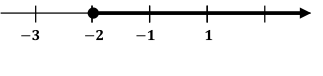# Free SAT Practice Questions

Question 1 of 1
ID: SAT-HOA-4
Section: Math - Heart of Algebra
Topics: Heart of Algebra; Inequality
Difficulty level: Hard

(Practice Mode: Single selected Question » Back to Overview)

Numeric Entry (Student-produced response) question$4\left(2x-1\right)-3x\le kx-2$

What is the value of the constant $k$ that satisfies the given inequality whose solution is shown in the line diagram above? (without calculator)